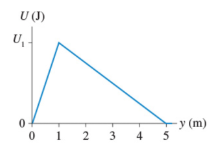# Problem: A system in which only one particle can move has the potential energy shown below. Suppose U1 = 88 JA. What is the y-component of the force on the particle at y = 0.5 m? B. What is the y-component of the force on the particle at y = 4 m?

###### FREE Expert Solution###### Problem Details

A system in which only one particle can move has the potential energy shown below. Suppose U= 88 JA. What is the y-component of the force on the particle at y = 0.5 m?

B. What is the y-component of the force on the particle at y = 4 m?